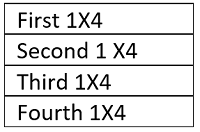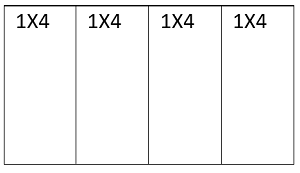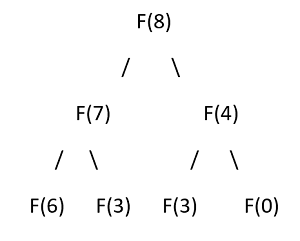Q:

# Number of ways to construct the grid

There is a grid with size NX4 and you have only one type of tiles to construct the grid. You can place either vertically or horizontally. You need to find, how many ways you can construct a grid of size N x 4 using the 1X4 tiles.

```    Input:
Input contains only one line with the value of N.

Output:
Print number of possible ways.

Constraints:
1 ≤ N ≤ 80
```

Example:

```    Input:
1

Output:
1

Explanation:
So n=1 and grid is 1X4. That’s why only one way we can build

Input:
4

Output:
2

Explanation:
So, n=4 and grid is 4X4. That's why two way we can build

First way is to place all four tiles horizontally one after one
Like below,Second way is to place all four tiles vertically one after one

Input:
5

Output:
3

Explanation:
So, n=5 and grid is 5X4. That's why two way we can build
First way is to place all five tiles horizontally one after one
Second way is, place four tiles vertically and place the last
tiles horizontally above them
Third way is, place the first tile horizontally and place other
four tiles vertically on that.```

The problem is recursive by nature and thus can be solved recursively.

Let,

```    f(n)=Total number of ways of constructing the grid
```

So, obviously f(n) would be

f(n)=f(n-1) +f(n-4) as we can either place last tile horizontally on the previous tiles or we can construct by vertically placing 4 1X4 blocks.

So let's say f(8)So on...

So, there are so many overlapping sub-problems and that's why we need to store and use Dynamic programming.

So, if we convert it into a DP solution,

```    1)  Declare dp[n+1] to store results for input n.
2)  Initialize dp=1,dp=1,dp=1,dp=1;
3)  for i=4 to n
dp[i]=dp[i-1]+dp[i-4];
4)  Return dp[n];
```

So dp[n] is the final result.

C++ Implementation:

``````#include <bits/stdc++.h>
using namespace std;

int main()
{
int n, item;

cout << "Enter value of n" << endl;
cin >> n;

long long int* dp = (long long int*)(malloc(sizeof(long long int) * (n + 1)));

dp = 1;
dp = 1;
dp = 1;
dp = 1;

for (int i = 4; i <= n; i++)
dp[i] = dp[i - 1] + dp[i - 4];

cout << "Number of ways to make the tile is: " << dp[n] << endl;

return 0;
}
``````

Output

```Enter value of n
5
Number of ways to make the tile is: 3```